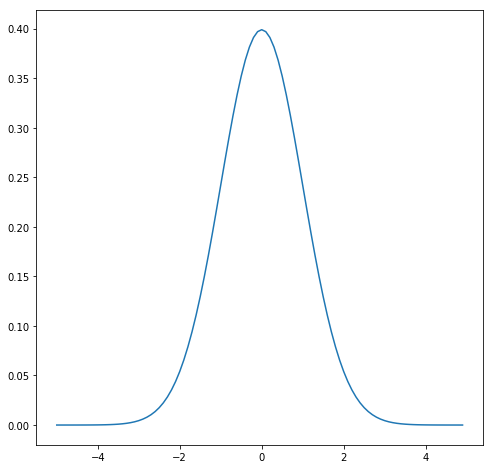Intro to OpenCV with Python

To work with OpenCV from python, you need to install it first:

pip install opencv-python

After we import cv2 we can directly work with images like so:

import cv2

The Normal Distribution

Diving deeper into data science I started to brush up my knowledge about math especially statistics.

The Mother of all DistributionsThe normal distribution was formulated by Carl Friedrich Gauß in 18XX and can be implemented in Python like the following :

def normal_distribution(x, mu=0, sigma=1):
sqrt_two_pi = math.sqrt(2*math.pi)
return math.exp(-(x-mu)**2 / 2 / sigma**2) / sqrt_two_pi * sigma